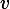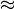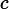# Planetary orbits viewed by a relativistic observer

• Ndaren

#### Ndaren

Suppose an observer flies through our solar system at ##v \approx c## relative to the Sun, such that he sees each planet's (and the sun's) mass as greatly increased. What happens to the orbits of the planets? Since he sees the center of mass of the solar system as flying past him at ##v \approx c##, do the orbits appear Newtonian to him or not?

Can we still apply other SR transformations (length contraction, velocity transformation) to the sun and planets and their orbits, given that their enormous mass/momentum should produce very strong gravity? If so, then

$u_{||} = \frac{u_{||}' + v}{1 + vu_{||}'/c^2} \Rightarrow u_{||} - v = \frac{u_{||}(1-\frac{v^2}{c^2})}{1 + vu_{||}'/c^2} \to 0$

$u_{\perp} = \frac{u_{\perp}'}{\gamma(1 + vu_{||}'/c^2})$

seem to imply that the observed orbital velocities of the planets relative to the sun will be less than their orbital velocities in the Sun's frame. How do we reconcile this with the much greater apparent mass of the sun, and the length contracted orbits on top of that? Shouldn't the planets spiral in towards the sun/each other?

he sees each planet's (and the sun's) mass as greatly increased

The "mass" that he sees as greatly increased is relativistic mass, but relativistic mass is not the source of gravity. The source of gravity is the stress-energy tensor, which is covariant; the Sun's effect on the planets is the same in all frames. This should make sense to you physically: you can't change the orbits of the planets by flying past the solar system very fast.

Since he sees the center of mass of the solar system as flying past him atv \approx c, do the orbits appear Newtonian to him or not?

To a good approximation, the orbits will appear as Lorentz transformed versions of the Newtonian orbits. I.e., they will still be closed orbits, but their shapes will differ because of Lorentz contraction.

The reason the above is an approximation is that the transformation being described is not actually a global Lorentz transformation, since there is no such thing in curved spacetime. But the spacetime curvature in the solar system is small enough that the transformation can be thought of for this purpose as a global Lorentz transformation, to a good approximation.

The "mass" that he sees as greatly increased is relativistic mass, but relativistic mass is not the source of gravity.
How can the relativistic mass not contribute to gravity? The observer will see the planets as having very large momentum/kinetic energy, which will contribute to the stress-energy tensor, and therefore to gravity (as this paper implies: http://arxiv.org/PS_cache/gr-qc/pdf/9909/9909014v1.pdf).

Thanks for the clarification regarding the form of the orbits.

How can the relativistic mass not contribute to gravity? The observer will see the planets as having very large momentum/kinetic energy, which will contribute to the stress-energy tensor, and therefore to gravity (as this paper implies: http://arxiv.org/PS_cache/gr-qc/pdf/9909/9909014v1.pdf).

You're looking for the GR solution to the gravitational field of rapidly moving massive bodies. That doesn't look anything like what you get if you try plugging the relativistic mass into the Newtonian formula for gravitational force, so if you use the Newtonian formula and then try using classical methods to calculate the orbits you are pretty much guaranteed bogus results.

The observer will see the planets as having very large momentum/kinetic energy, which will contribute to the stress-energy tensor

Yes, but how do they contribute?

Consider the simpler case of the energy-momentum 4-vector. Energy and momentum both contribute to that, yes, so an object moving at close to the speed of light has an energy much larger than its rest mass and a correspondingly large momentum. But the invariant (squared) length of that 4-vector is energy squared minus momentum squared, which is just the (squared) invariant mass, and is the same in all frames.

The behavior of the stress-energy tensor is a bit more complicated because it's a tensor, not a 4-vector, so it doesn't have a single invariant "length". But the general idea is the same: changing frames changes the energy and momentum, but their contribution to gravity works similarly to their contribution to invariant mass, i.e., like the large momentum being subtracted from the large energy, not added to it.

as this paper implies

That paper is making a different argument; it is saying that if you take a system composed of particles in motion, the gravity produced by such a system includes a contribution from the kinetic energy of the particles in the center of mass frame of the system. In other words, if I have a box of gas and I heat it up, the gravity produced by the box increases, because heating it up means increasing the kinetic energy of the particles in the gas in the rest frame of the box. That's not at all the same as leaving the box unchanged but moving relative to it at high speed.

Note that if you're going to take a Special Relativity approximation, the whole system is massively time-dilated. If I remember correctly, energy and forces both effectively increase by the time dilation factor due to the velocity, but acceleration decreases by the square of the same factor.

The "mass" that he sees as greatly increased is relativistic mass, but relativistic mass is not the source of gravity. The source of gravity is the stress-energy tensor, which is covariant; the Sun's effect on the planets is the same in all frames. This should make sense to you physically: you can't change the orbits of the planets by flying past the solar system very fast.

What do you mean by the stress-energy tensor being covariant? Probably the tensor will have changed components, because it's a rank-2 tensor [and transforms accordingly].
The Einstein equations remain covariant...

What do you mean by the stress-energy tensor being covariant?

This:

it's a rank-2 tensor [and transforms accordingly].

:)
This

OK... sorry, I misunderstood "covariant" in this context [because sometimes covariant are called the equations that don't change]

sometimes covariant are called the equations that don't change

They do if you write them down component by component, since, as you noted, the components change. But the whole set of equations has to have well-defined transformation properties, which means both sides have to be the same kind of geometric object.

In the case of the Einstein Field Equation, both sides are symmetric 2nd-rank tensors. This means the field "equation" is actually ten equations (since symmetric 2nd-rank tensors have ten independent components), each of which, individually, can change when you change coordinates. But the whole set of equations has to transform like an equation relating two symmetric 2nd-rank tensors.

The whole set of stuff I just said is, properly speaking, what the term "covariant" refers to. Sometimes one focuses more on one aspect than another.

Although is it legit to use Special Relativity terminology to explain how things behave near the velocity of light?
Obviously the solar system is not a local region... (local Lorentz covariance of GR)

The observer will see the planets as having very large momentum/kinetic energy, which will contribute to the stress-energy tensor, and therefore to gravity (as this paper implies: http://arxiv.org/PS_cache/gr-qc/pdf/9909/9909014v1.pdf).
The paper implies no such thing. The paper discusses the gravitational mass of systems with internal kinetic energy, but no momentum. It is important to not over generalize the analysis of a paper.

The 1985 Olson and Guarino paper "Measuring the active gravitational mass of a moving object" has one of the better analysis of the effects of a flyby by a gravitating object and is frequently cited here on PF.

From the abstract:

If a heavy object with rest mass M moves past you with a velocity comparable to the speed of light, you will be attracted gravitationally towards its path as though it had an increased mass. If the relativistic increase in active gravitational mass is measured by the transverse (and longitudinal) velocities which such a moving mass induces in test particles initially at rest near its path, then we find, with this definition, that M rel=γ(1+β2)M. Therefore, in the ultrarelativistic limit, the active gravitational mass of a moving body, measured in this way, is not γM but is approximately 2γM.

Note that the way specified in the abstract above of measuring "gravitational mass" is more or less pedagogical, but the the setup is simple enough to describe and analyze easily. Furthermore, if one finds and reads the whole paper there is some discussion of some of other ways of talking about "mass" in general relativity, such as the Bondi and ADM masses.

Although is it legit to use Special Relativity terminology to explain how things behave near the velocity of light?
Obviously the solar system is not a local region... (local Lorentz covariance of GR)

For what happens when seen from a rapidly moving viewpoint compared with a static viewpoint, you can use Special Relativity and Lorentz transformations as a very good approximation in most cases.